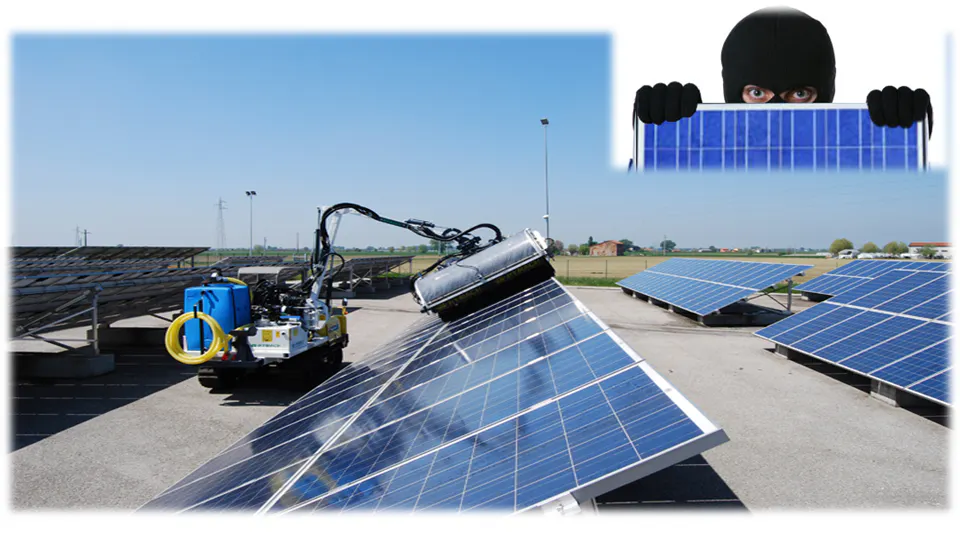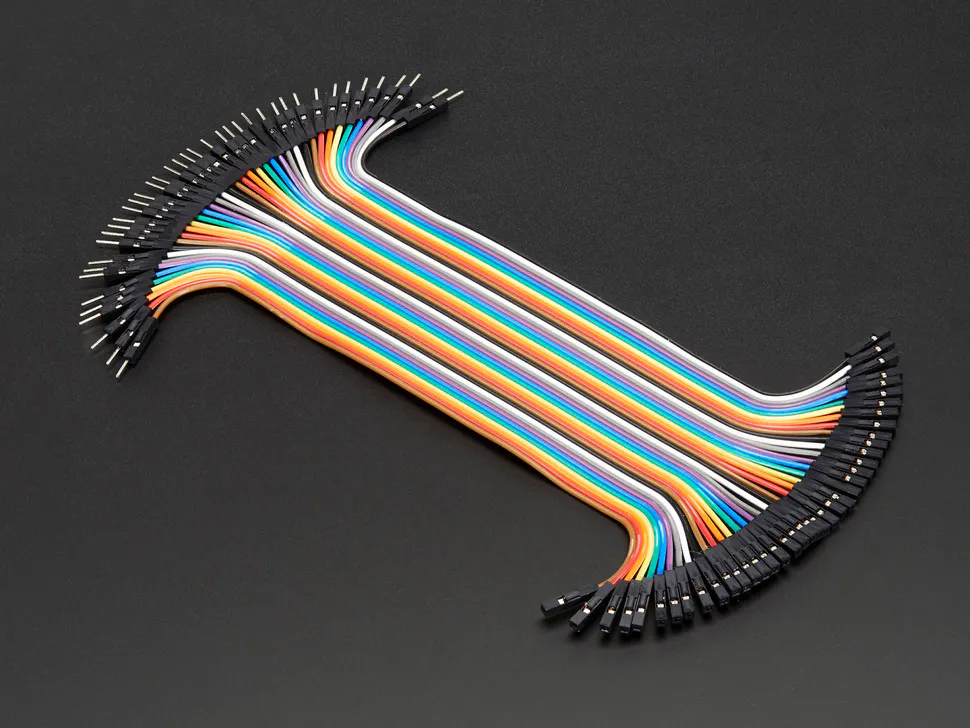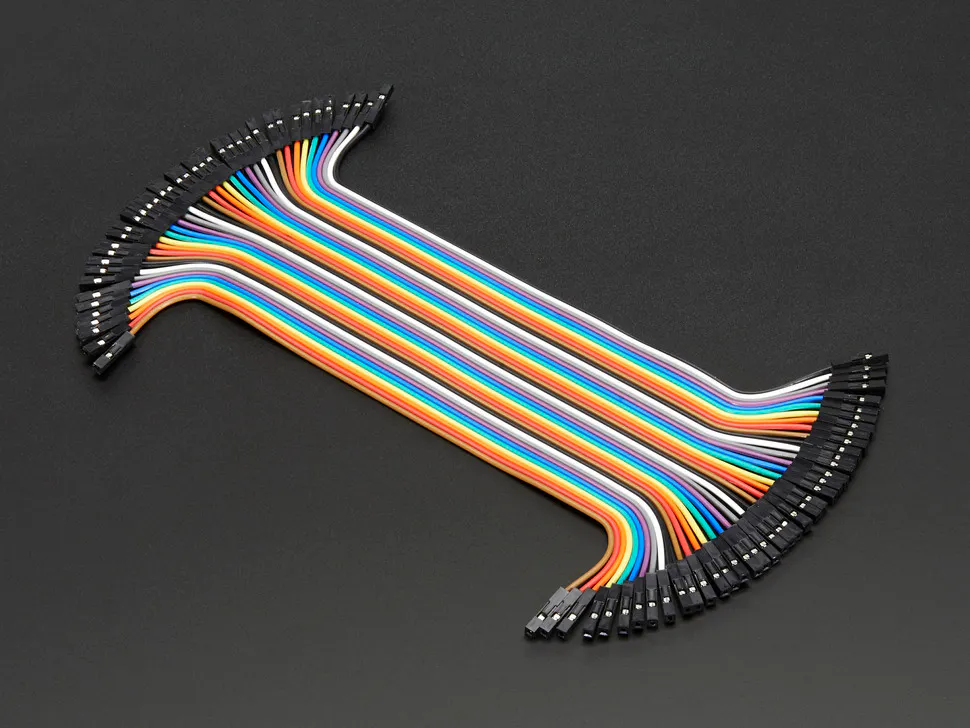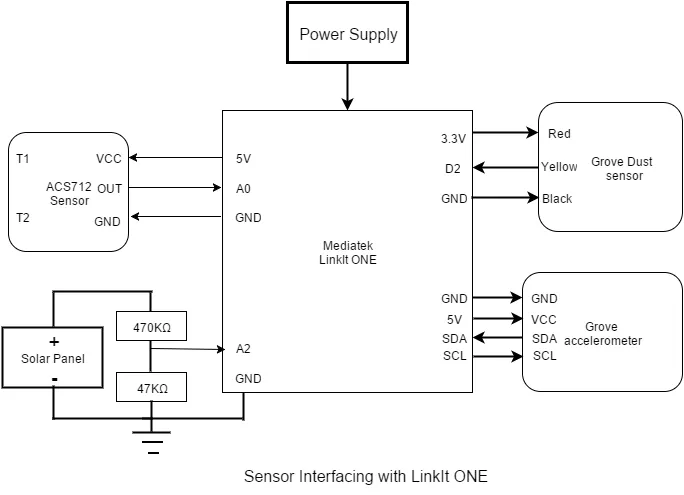# Solar Panel Dual Management System (SP DMS)

This IoT prototype performs two functions: 1) Theft prevention and 2) Maintenance Indication of solar panel using LinkIt ONE and Sensors

IntermediateFull instructions provided3 days13,696## Things used in this project

### Hardware components

 Grove Dust Sensor
×1
 Grove 3-axis accelerometer sensor
×1
 ACS712 current sensor module (5A)
×1
 Resistors 47k
×1
 Resistor 470k
×1
 Male/Male Jumper Wires Components mention after this needed only if you build base shield
×1Male/Female Jumper Wires
×1Female/Female Jumper Wires
×1
 General Purpose PCB
×1
 2.54mm pitch pin headers - Female 40pin in 1 line
×1
 2.54mm pitch 40 pin male header strip
×1
 2pin Screw terminal
×1

### Software apps and online servicesArduino IDEUbidots

### Hand tools and fabrication machines

 MultimeterSoldering iron (generic) Required only when making base shield
 PCB cutter Required only when making base shield

## Schematics

### Sensors Interfacing Block Diagram### Sensors Interfacing Block Diagram## Code

### Theft_protection_accelerometer.ino

Arduino
```#include <Wire.h>      //library to establised I2C communication between LinkIt One and Grove - 3-Axis Digital Accelerometer(16g)

int x_initial,y_initial,z_initial;
double xyz_initial;
double ax_initial,ay_initial,az_initial;

//Function to Store initial axis value of x,y & z for comparision
void axis_initialization(){
ax_initial = xyz_initial;
ay_initial = xyz_initial;
az_initial = xyz_initial;
delay(500);
}

//To compare initial values with current reading of x,y & z values for theft protection
int compareResult(){
//Boring accelerometer stuff
int x,y,z,Xchange,Ychange,Zchange;
// Output x,y,z values

Serial.print("values of X , Y , Z: ");
Serial.print(x);
Serial.print(" , ");
Serial.print(y);
Serial.print(" , ");
Serial.println(z);

double xyz;
double ax,ay,az;
ax = xyz;
ay = xyz;
az = xyz;

Serial.print("X=");
Serial.print(ax);
Serial.println(" g");
Serial.print("Y=");
Serial.print(ay);
Serial.println(" g");
Serial.print("Z=");
Serial.println(az);
Serial.println(" g");
Serial.println("**********************");

if((int)ax!=(int)ax_initial){
Xchange=1;
}
else {
Xchange=0;
}

Serial.print("Xchange:");
Serial.println(Xchange);

if((int)ay!=(int)ay_initial){
Ychange=1;
}
else {
Ychange=0;
}

Serial.print("Ychange:");
Serial.println(Ychange);

if((int)az!=(int)az_initial){
Zchange=1;
}
else {
Zchange=0;
}

Serial.print("Zchange:");
Serial.println(Zchange);

if((Xchange==1)||(Ychange==1)||(Zchange==1)){
return 1;
}
else return 0;

}
void setup(){
Serial.begin(9600);
delay(5000);
axis_initialization();
Serial.println("SP DMS");
}

void loop(){
int unauthorised_activity;
unauthorised_activity=compareResult();
if(unauthorised_activity==1){
Serial.println("There is unauthorised activity...");
}
delay(500);

}
```

### Grove_dust_sensor.ino

Arduino
```/*
Interface to Shinyei Model PPD42NS Particle Sensor
Program by Christopher Nafis
Written April 2012

http://www.seeedstudio.com/depot/grove-dust-sensor-p-1050.html
http://www.sca-shinyei.com/pdf/PPD42NS.pdf

JST Pin 1 (Black Wire)  => Arduino GND
JST Pin 3 (Red wire)    => Arduino 5VDC
JST Pin 4 (Yellow wire) => Arduino Digital Pin 8
*/

int pin = 2;
unsigned long duration;
unsigned long starttime;
unsigned long sampletime_ms = 30000;
unsigned long lowpulseoccupancy = 0;
float ratio = 0;
float concentration = 0;

void setup() {
Serial.begin(9600);
pinMode(2,INPUT);
starttime = millis();
}

void loop() {
duration = pulseIn(pin, LOW);
lowpulseoccupancy = lowpulseoccupancy+duration;

if ((millis()-starttime) > sampletime_ms)
{
ratio = lowpulseoccupancy/(sampletime_ms*10.0);  // Integer percentage 0=>100
concentration = 1.1*pow(ratio,3)-3.8*pow(ratio,2)+520*ratio+0.62; // using spec sheet curve
Serial.print(lowpulseoccupancy);
Serial.print(",");
Serial.print(ratio);
Serial.print(",");
Serial.println(concentration);
lowpulseoccupancy = 0;
starttime = millis();
}
}
```

Arduino
```uint32_t analogPin = A0;
uint32_t value = 0;

float vout = 0.0;
float vin = 0.0;
float R1 = 470000.0;// resistance of R1 (470k)
float R2 = 47000.0; // resistance of R2 (47K)

void setup()
{
Serial.begin(9600);
}
void loop()
{
Serial.print("Analog Value =");
Serial.println(value);
vout = (value * 5.0) / 1024.0;
vin = vout*(R1+R2)/R2;
Serial.print("Voltage =");
Serial.println(vin);
delay(2000);
}
```

Arduino
```uint32_t analogPin = A2;
uint32_t value = 0;

float vout = 0.0;
float vin = 0.0;
float R1 = 470000.0; // resistance of R1 (470k)
float R2 = 47000.0; // resistance of R2 (47K)

void setup()
{
Serial.begin(9600);
}
void loop()
{
float avgVolt=0,clb;
for(int i=0;i<100;i++){
vout = (value * 5.0) / 1024.0;
vin = vout*(R1+R2)/R2;
clb=vin*0.767;
vin=vin+clb;
avgVolt=avgVolt+vin;
}
avgVolt=avgVolt/100.0;
Serial.print("Voltage =");
Serial.println(avgVolt,1);

}
```

### current_sensor_test.ino

Arduino
```//Code to measure current AC/DC using ACS712 sensor module(5A model)
// the setup routine runs once when you press reset:
void setup() {
Serial.begin(9600);    // initialize serial communication at 9600 bits per second:
}

// the loop routine runs over and over again forever:
void loop() {
float average = 0;
for(int i = 0; i < 1000; i++) {
average = average + (.0264 * analogRead(A0)-13.51);
delay(1);
}
Serial.print(average);
Serial.println("mA");
}
```

Arduino
Combine test code for current and voltage.
```uint32_t value = 0;

float vout = 0.0;
float vin = 0.0;
float R1 = 470000.0; // resistance of R1 (470k)
float R2 = 47000.0; // resistance of R2 (47K)

// the setup routine runs once when you press reset:
void setup() {
Serial.begin(9600);    // initialize serial communication at 9600 bits per second:
}

// the loop routine runs over and over again forever:
void loop() {
float avgCurrent = 0,avgVolt=0,clb;
for(int i = 0; i < 1000; i++) {
avgCurrent = avgCurrent + (.0264 * analogRead(A0)-13.51);
delay(1);
}
Serial.print("  Current =");
Serial.print(avgCurrent);
Serial.print("mA");
for(int i=0;i<100;i++){
vout = (value * 5.0) / 1024.0;
vin = vout*(R1+R2)/R2;
clb=vin*0.767;
vin=vin+clb;
avgVolt=avgVolt+vin;
}
avgVolt=avgVolt/100.0;
Serial.print("\tVoltage =");
Serial.println(avgVolt,1);
}
```

Arduino
All sensors combine test code.
```#include <Wire.h>      //library to establised I2C communication between LinkIt One and Grove - 3-Axis Digital Accelerometer(16g)

//Accelerometer variables

//variables to store the initial state of device after installation when power on
int x_initial,y_initial,z_initial;
double xyz_initial;
double ax_initial,ay_initial,az_initial;

uint32_t Volt_value = 0;
float vout = 0.0;
float vin = 0.0;
float R1 = 470000.0; // resistance of R1 (470k)
float R2 = 47000.0; // resistance of R2 (47K)

//variables for Dust Sensor
int pin = 2;
unsigned long duration;
unsigned long starttime;
unsigned long sampletime_ms = 30000;
unsigned long lowpulseoccupancy = 0;
float ratio = 0;
float concentration = 0;

//Function to Store initial axis value of x,y & z for comparision
void axis_initialization(){
ax_initial = xyz_initial;
ay_initial = xyz_initial;
az_initial = xyz_initial;
delay(500);
}

//To compare initial values with current reading of x,y & z values for theft protection
int compareResult(){
//Boring accelerometer stuff
int x,y,z,Xchange,Ychange,Zchange;
// Output x,y,z values
double xyz;
double ax,ay,az;
ax = xyz;
ay = xyz;
az = xyz;

if((int)ax!=(int)ax_initial){
Xchange=1;
}
else {
Xchange=0;
}

if((int)ay!=(int)ay_initial){
Ychange=1;
}
else {
Ychange=0;
}

if((int)az!=(int)az_initial){
Zchange=1;
}
else {
Zchange=0;
}

if((Xchange==1)||(Ychange==1)||(Zchange==1)){
return 1;
}
else return 0;
}

void DustCal(){
duration = pulseIn(pin, LOW);
lowpulseoccupancy = lowpulseoccupancy+duration;

if ((millis()-starttime) > sampletime_ms)
{
ratio = lowpulseoccupancy/(sampletime_ms*10.0);  // Integer percentage 0=>100
concentration = 1.1*pow(ratio,3)-3.8*pow(ratio,2)+520*ratio+0.62; // using spec sheet curve
Serial.print("  lowpulseoccupancy: ");
Serial.print(lowpulseoccupancy);
Serial.print(",");
Serial.print("ratio: ");
Serial.print(ratio);
Serial.print(",");
Serial.print("concentration: ");
Serial.println(concentration);
lowpulseoccupancy = 0;
starttime = millis();
}
Serial.println();
}

void setup(){
Serial.begin(9600);
axis_initialization();
pinMode(2,INPUT);
starttime = millis();
}

void loop(){
int unauthorised_activity=0;
unauthorised_activity=compareResult();

if(unauthorised_activity==1){
Serial.println("There is unauthorised activity...");
}
else {
float avgCurrent = 0,avgVolt=0,clb=0;

for(int i = 0; i < 1000; i++) {
avgCurrent = avgCurrent + (.0264 * analogRead(A0)-13.51);
delay(1);
}
Serial.print("  Current =");
Serial.print(avgCurrent);
Serial.print("mA");
for(int i=0;i<100;i++){
vout = (Volt_value * 5.0) / 1024.0;
vin = vout*(R1+R2)/R2;
clb=vin*0.767;
vin=vin+clb;
avgVolt=avgVolt+vin;
}
avgVolt=avgVolt/100.0;
Serial.print("\tVoltage =");
Serial.print(avgVolt,1);
Serial.print("V");
Serial.print("\tPower =");
Serial.print((avgCurrent/1000.0)*avgVolt);
Serial.println("W");

DustCal();

}
delay(500);
}
```

### index.html

HTML
```<!DOCTYPE html>
<html>

<title>Solar Panel Dual Management System</title>
<meta charset="utf-8">
<style>
body {
background: #4285F4;
background-size:100%;
}
h1{
color: #ffffff;
}
.container{
color: #ff0000;
}
</style>
<body>
<h1  align="center">Solar Panel Dual Management System</h1>
</div>
<div class="container" align="right">
<a href="location.html"><button type="button">Track Panel</button></a>
</div>
<div class="SensorsData" align="center">
<iframe width="430" height="280" frameborder="0" src="https://app.ubidots.com/ubi/getchart/lfBlDRazzsEpkEs6urLmZtUG008" style="background: transparent"></iframe>&nbsp&nbsp&nbsp&nbsp
<iframe width="430" height="280" frameborder="0" src="https://app.ubidots.com/ubi/getchart/SQHxvj5U7t2IrQC6rROd9HfGNdo" style="background: transparent"></iframe>
<br><br>
<iframe width="430" height="280" frameborder="0" src="https://app.ubidots.com/ubi/getchart/xxw39mZOOQ9xs1skwdAUBx-HAh0" style="background: transparent"></iframe>&nbsp&nbsp&nbsp&nbsp
<iframe width="430" height="280" frameborder="0" src="https://app.ubidots.com/ubi/getchart/mL0s_GCoL-qGQl0-9kZeSp3YLn4" style="background: transparent"></iframe>
<br><br>
<iframe width="430" height="280" frameborder="0" src="https://app.ubidots.com/ubi/getchart/7Cz3vWe5dorePxvA-sich25LJk4" style="background: transparent"></iframe>
</div>

</body>
</html>
```

### location.html

HTML
```<!DOCTYPE html>
<html>

<title>Solar Panel Dual Management System</title>
<style>
body {
background: #4285F4;
background-size:100%;
}
h1{
color: #ffffff;
}
h2{
color: #ffffff;
}
</style>
<body>
<h1  align="center">Solar Panel Dual Management System</h1>
</div>
<div class="container" align="left">
<a href="index.html"><button type="button">Back</button></a>
</div>
<div class="SensorsData" align="center">
<iframe width="870" height="480" frameborder="0" src="https://app.ubidots.com/ubi/getchart/Em9WiAc-RENRvLlgtYbR2-9uSYo" style="background: transparent"></iframe>
</div>
<h2  align="center">GPS Location Of Solar Panel</h2>
</body>
</html>
```

### Project_Code.ino

Arduino
Final working project code. For simplicity I have divided major section of codes in various function which will help you to understand the flow of program.
```/********************************************************************************************************************************************/
/*  During normal operation when no theft occurs LinkIt ONE sends the value of curren,voltage,dust sensor and battery value only            */
/*  Once there is unauthorized activity happens then there is change in accelerometer value and sense by LinkIt,                            */
/*  After this LinkIt ONE sends the GPS location and Battery value to server and alert is indicated by email                                */
/********************************************************************************************************************************************/
#include <LGPS.h>         // LinkIt GPS Library
#include <LBattery.h>     // Want to be able to read the battery charge level
#include <LGPRS.h>        //include the base GPRS library
#include <LGPRSClient.h>  //include the ability to Post and Get information using HTTP
#include <Wire.h>         //library to establised I2C communication between LinkIt One and Grove - 3-Axis Digital Accelerometer(16g)

// These are the variables you will want to change based on your IOT data streaming account / provider
#define URL     "things.ubidots.com"
#define TOKEN   "MuIKgAEIYR4AxCeaWEpsPAxKHmopskmGxM1k45mPrgeQSS0pZuKrXXi0zhse"   //replace with your Ubidots token generated in your profile tab
#define voltID  ""       //create a Voltage sensor variable in Ubidots and put its ID here
#define ampsID  ""       //create a Current sensor variable in Ubidots and put its ID here
#define dustID  ""       //create a Dust sensor variable in Ubidots and put its ID here
#define alertID ""       //create a Alert variable in Ubidots and put its ID here
#define batID   ""       //create a Battery variable in Ubidots and put its ID here

// Create instantiations of the GPRS and GPS functions
LGPRSClient globalClient;    // See this support topic from Mediatek - http://labs.mediatek.com/forums/posts/list/75.page
gpsSentenceInfoStruct info;  // instantiate
String Location = "";        //variable to GPS location latitude and longitude in string form

//Create instantiations of the ADXL345 Accelerometer function

//variables to store the initial state of device after installation when power on
int x_initial,y_initial,z_initial;
double xyz_initial;
double ax_initial,ay_initial,az_initial;

//variables for Voltage and current reading
uint32_t Volt_value = 0;
float vout = 0.0;
float vin = 0.0;
float R1 = 470000.0;                   // resistance of R1 (470k)
float R2 = 47000.0;                    // resistance of R2 (47K)
float avgCurrent = 0,avgVolt=0,clb=0;

//variables for Dust Sensor
int pin = 2;
unsigned long duration;
unsigned long starttime;
unsigned long sampletime_ms = 30000;
unsigned long lowpulseoccupancy = 0;
float ratio = 0;
float concentration = 0;

//function to send data of sensors on webserver
String value = String(LBattery.level());  //calculating the level of battery
String payload;                           //Variable to collect all sensor data for data upload on Webserver
String le;                                //length of the payload in characters
if(alert==1){                              //enters when there is theft
LGPS.getData(&info);                   // Get a GPS fix
if (ParseLocation((const char*)info.GPGGA)) {    // This is where we break out needed location information
Serial.print("Location is: ");
Serial.println(Location);                      // This is the format needed by Ubidots
}
if (Location.length()==0) {                      //enters when no GPS location available otherwise execute else case
payload = "[{\"variable\":\"" alertID "\",\"value\":" + String(alert) + "},{\"variable\":\"" batID "\",\"value\":"+ value + "}]";  //Build the JSON packet without GPS info// with GPS info
}
else {
payload = "[{\"variable\":\"" alertID "\",\"value\":" + String(alert) + "},{\"variable\":\"" batID "\",\"value\":"+ value + ", \"context\":"+ Location + "}]"; //Build the JSON packet with GPS info
}

}
else {
payload = "[{\"variable\":\"" ampsID "\",\"value\":"+ String(avgCurrent)+"},{\"variable\":\"" voltID "\",\"value\":" + String(avgVolt) + "},{\"variable\":\"" dustID "\",\"value\":" + String(concentration) + "},{\"variable\":\"" alertID "\",\"value\":" + String(alert) + "},{\"variable\":\"" batID "\",\"value\":"+ value + "}]";
}

// if you get a connection, report back via serial:
Serial.print("Connect to ");
Serial.println(URL);
if (globalClient.connect(URL, 80)){
// Build HTTP POST request
Serial.println("connected");
globalClient.print(F("POST /api/v1.6/collections/values/?token="));
globalClient.print(TOKEN);
globalClient.println(F(" HTTP/1.1"));
globalClient.println(F("Content-Type: application/json"));
globalClient.print(F("Content-Length: "));
globalClient.println(le);
globalClient.print(F("Host: "));
globalClient.println(URL);
globalClient.println();
globalClient.println();
globalClient.println((char)26);   //This terminates the JSON SEND with a carriage return
}
else{
// if you didn't get a connection to the server:
Serial.println("connection failed");
}
delay(100);

// if there are incoming bytes available
// from the server, read them and print them:
if (globalClient.available()){
Serial.print(c);
}

// if the server's disconnected, stop the globalClient:
if (!globalClient.available() && !globalClient.connected()){
Serial.println();
Serial.println("disconnecting.");
globalClient.stop();
}

}

boolean ParseLocation(const char* GPGGAstr){
// Refer to http://www.gpsinformation.org/dale/nmea.htm#GGA
// Sample data: \$GPGGA,123519,4807.038,N,01131.000,E,1,08,0.9,545.4,M,46.9,M,,*47

char latarray;
char longarray;
int index = 0;
Serial.println(GPGGAstr);
Serial.print("Fix Quality: ");
Serial.println(GPGGAstr);

if (GPGGAstr=='0') {         //  This is the place in the sentence that shows Fix Quality 0 means no fix
Serial.println("No GPS Fix");
Location = "";                 // No fix then no Location string
return 0;
}

String GPSstring = String(GPGGAstr);
for (int i=20; i<=26; i++) {         // We have to jump through some hoops here
latarray[index] = GPGGAstr[i];     // we need to pick out the minutes from the char array
index++;
}

float latdms = atof(latarray);               // and convert them to a float
float lattitude = latdms/60;                 // and convert that to decimal degrees
String lattstring = String(lattitude);       // Then put back into a string
Location = "{\"lat\":";
if(GPGGAstr == 'S') Location = Location + "-";
Location += GPSstring.substring(18,20) + "." + lattstring.substring(2,4);
index = 0;

for (int i=33; i<=38; i++) {           // And do the same thing for longitude
longarray[index] = GPGGAstr[i];      // the good news is that the GPS data is fixed column
index++;
}
float longdms = atof(longarray);       // and convert them to a float
float longitude = longdms/60;          // and convert that to decimal degrees
String longstring = String(longitude); // Then put back into a string
Location += " ,\"lng\":";

if(GPGGAstr == 'W') Location = Location + "-";
if(GPGGAstr == '0') {
Location = Location + GPSstring.substring(31,33) + "." + longstring.substring(2,4) + "}";
}
else {
Location = Location + GPSstring.substring(30,33) + "." + longstring.substring(2,4) + "}";
}
return 1;
}

//Function to Store initial axis value of x,y & z for comparision
void axisInitialization(){
ax_initial = xyz_initial;
ay_initial = xyz_initial;
az_initial = xyz_initial;
delay(500);
}

//To compare initial values with current reading of x,y & z axis values for theft protection
int compareResult(){
//Boring accelerometer stuff
int x,y,z,Xchange,Ychange,Zchange;
// Output x,y,z values
double xyz;
double ax,ay,az;
ax = xyz;
ay = xyz;
az = xyz;

if((int)ax!=(int)ax_initial){
Xchange=1;
}
else {
Xchange=0;
}

if((int)ay!=(int)ay_initial){
Ychange=1;
}
else {
Ychange=0;
}

if((int)az!=(int)az_initial){
Zchange=1;
}
else {
Zchange=0;
}

if((Xchange==1)||(Ychange==1)||(Zchange==1)){
return 1;
}
else return 0;
}

//Function to calculate voltage, current and power of Solar Panel for Efficieny test
void powerCal(){
avgCurrent = 0,avgVolt=0,clb=0;
for(int i = 0; i < 1000; i++) {
avgCurrent = avgCurrent + (.0264 * analogRead(A0)-13.51);
delay(1);
}
Serial.print("  Current =");
Serial.print(avgCurrent);
Serial.print("mA");
for(int i=0;i<100;i++){
vout = (Volt_value * 5.0) / 1024.0;
vin = vout*(R1+R2)/R2;
clb=vin*0.767;
vin=vin+clb;
avgVolt=avgVolt+vin;
}
avgVolt=avgVolt/100.0;
Serial.print("\tVoltage =");
Serial.print(avgVolt,1);
Serial.print("V");
Serial.print("\tPower =");
Serial.print((avgCurrent/1000.0)*avgVolt);                        //Computing power
Serial.println("W");
}

//Function for calculating the dust concentration level
void dustCal(){
duration = pulseIn(pin, LOW);                                       //reads the duration or total of low pulse on pin
lowpulseoccupancy = lowpulseoccupancy+duration;

if ((millis()-starttime) > sampletime_ms)
{
ratio = lowpulseoccupancy/(sampletime_ms*10.0);                   // Integer percentage 0=>100
concentration = 1.1*pow(ratio,3)-3.8*pow(ratio,2)+520*ratio+0.62; // using spec sheet curve
Serial.print("  lowpulseoccupancy: ");
Serial.print(lowpulseoccupancy);
Serial.print(",");
Serial.print("ratio: ");
Serial.print(ratio);
Serial.print(",");
Serial.print("concentration: ");
Serial.println(concentration);
lowpulseoccupancy = 0;
starttime = millis();
}
Serial.println();
}

//runs only once when power on or reset
void setup(){
Serial.begin(9600);                                  // setup Serial port

LGPS.powerOn();                                      // Start the GPS first as it takes time to get a fix
Serial.println("GPS Powered on, and waiting ...");
Serial.println("Attach to GPRS network");            // Attach to GPRS network - need to add timeout
while (!LGPRS.attachGPRS("","","")) {                //attachGPRS(const char *apn, const char *username, const char *password);
delay(500);
}
LGPRSClient client;                                  //Client has to be initiated after GPRS is established with the correct APN settings - see above link
globalClient = client;                               // Again this is a temporary solution described in support forums
delay(1000);
deviceLocation(0);                                   //Sending device location first time during installation and passing 0 means no theft

delay(3000);                                         //setup time so when power on no error in storing initial axis value of panel
axisInitialization();                                //calling function to store the intial installed axis of system

pinMode(2,INPUT);                                    //Initializing digital pin 2 as input for reading dust sensor value
starttime = millis();                                //Returns the number of milliseconds since the LinIt ONE board began running the current program
}

//forever loop code
void loop(){
int unauth_activity=0;
unauth_activity= compareResult();                         //Function to check or compare the axis value of panel for any change

if(unauth_activity==1){
Serial.println("There is unauthorised activity...");
deviceLocation_data(1);                               //Function for sending device location with battery level for tracing and passing 1, which is alert indication of theft
delay(100);
}
else {
powerCal();               //calling function for calculating voltage, current and power provided by solar panel
dustCal();                //calling function for calculating deposition of dust concentration on panel
deviceLocation_data(0);   //Function for sending sensor data for Maintenance Indication or monitoring of Solar panel for Efficiency check and passing 0 means normal acitvity
}
delay(500);
}
```

## Credits

### Brijesh Singh

23 projects • 35 followers
Utilizing the spare time to Make and Share DIY Electronics and IoT projects with the online maker community.